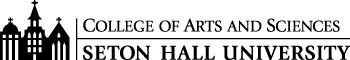# B.S. in Mathematics Curriculum

In addition to meeting the standards and requirements of the College of Arts and Sciences, degree candidates must complete additional requirements with the department of Mathematics and Computer Science. All programs are planned in consultation with a department adviser who may modify the program in view of the students' backgrounds and objectives. An asterisk (*) indicates strongly recommended courses.

Please note: Classes and program requirements are subject to change. Students who enroll at Seton Hall will follow the catalogue guidelines for the year they matriculate. Catalogues for each year are generally released in the late summer.

#### Freshman Year

CSAS 1114 Introduction of Program Design I 3
MATH 1501 Calculus I for Mathematical & Physical Sciences 4
MATH 1511 Calculus JI for Mathematical & Physical Sciences 4
MATH 1611 Introduction to Discrete Mathematics 3
PHIL 1204 Symbolic Logic* 3

#### Sophomore Year

MATH 2511 Calculus III for Mathematical & Physical Sciences 4
MATH 2711 Introduction to Probability and Statistics 4
MATH 2813 Linear Algebra 4

#### Junior Year - Senior Year

MATH 3515 Analysis 4
MATH 3626 Applied Matrix Techniques 3
MATH 3815 Abstract Algebra 4
MATH 3912 Junior Seminar 3
 MATH 3111 History of Mathematics 3 MATH 3411 (CSAS 3411) Graph Algorithms 3 MATH 3512 (CSAS 3513) Introduction to Numerical Analysis 3 MATH 3611 Introduction to Operations Research 3 MATH 3612 Discrete Mathematics 3 MATH 3614 Graph Theory 3 MATH 3711 Statistical Analysis 3 MATH 3721 Financial Calculus 3 MATH 3813 Number Theory 3 MATH 3911 Geometry 3 MATH 4512 Introduction to Complex Analysis 3 MATH 4516 Advanced Topics in Analysis 3 MATH 4712 Advanced Topics in Applied Prob and Stats 3 MATH 4816 Advanced Topics in Algebra 3 MATH 4911 Introduction to Topology 3 Total Credits 55
 MATH 4912 Senior Project 3